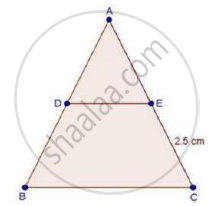Share

Books Shortlist
Your shortlist is empty

# Solution for In δAbc, D and E Are Points on the Sides Ab and Ac Respectively Such that De || Bc If "Ad"/"Bd"=4/5 And Ec = 2.5 Cm, Find Ae - CBSE Class 10 - Mathematics

ConceptBasic Proportionality Theorem Or Thales Theorem

#### Question

In ΔABC, D and E are points on the sides AB and AC respectively such that DE || BC

If "AD"/"BD"=4/5 and EC = 2.5 cm, find AE

#### SolutionWe have, DE || BC

Therefore, by basic proportionality theorem,

We have,

"AD"/"BD"="AE"/"EC"

rArr4/5="AE"/2.5

rArr"AE"=(4xx2.5)/5

⇒ AE = 2 cm

Is there an error in this question or solution?

#### Video TutorialsVIEW ALL 

Solution for question: In δAbc, D and E Are Points on the Sides Ab and Ac Respectively Such that De || Bc If "Ad"/"Bd"=4/5 And Ec = 2.5 Cm, Find Ae concept: Basic Proportionality Theorem Or Thales Theorem. For the course CBSE
S